JavaScript Booleans

# JavaScript Booleans

JavaScript Booleans
JavaScript Boolean returns a value either true or false.
The JavaScript Boolean Function
Boolean function is used to check if an expression or variable is true:
Example1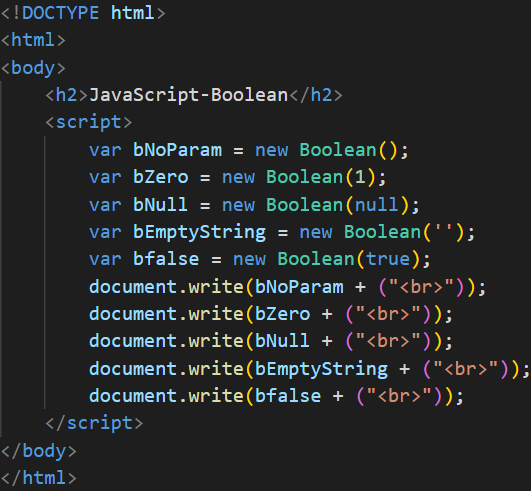Output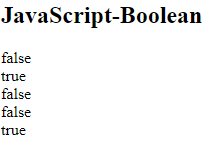Example2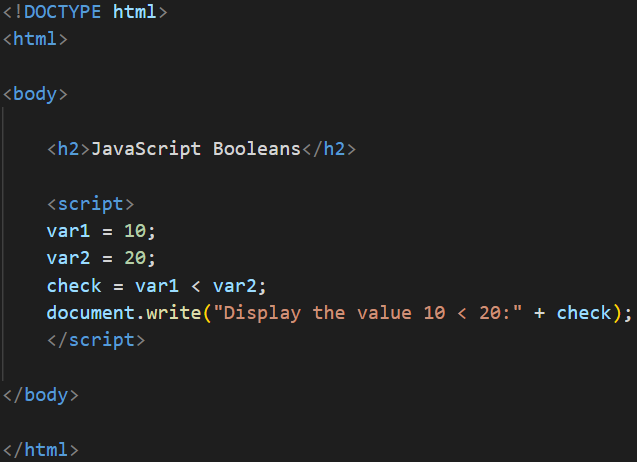Output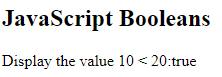JavaScript Comparison Operators
Comparison operators are used to compare two values.
The comparison operators are as shown below:
 Operator Example Description == a == b Equal to != a != b Not equal to > a > b Greater than < a < b Less than <= a <= b Less than equal to >= a >= b Greater than equal to
Example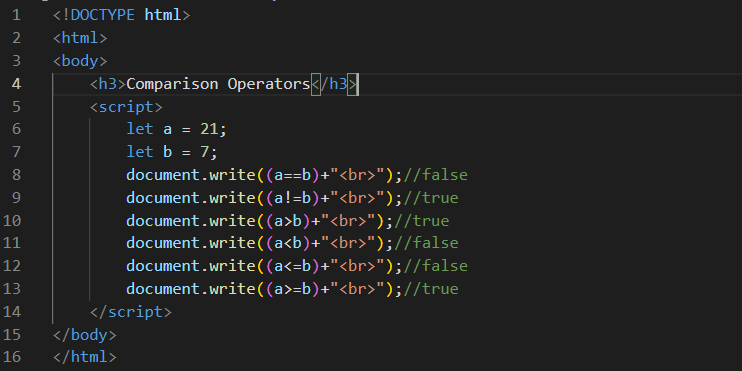Output
Comparison Operators
false
true
true
false
false
true